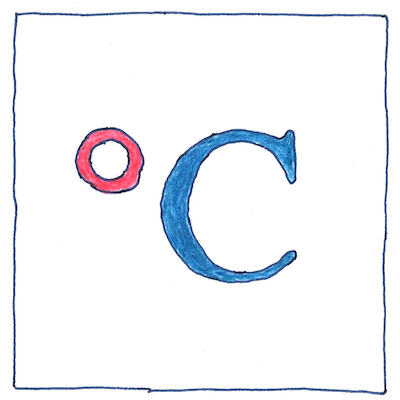# 1742

## The book of science

Tom Sharp

 Anders Celsius, Jean-Pierre Christin thermometry

• Anders Celsius realized
• the need for a standard temperature scale
• based on two scientifically reproducable points.
• He showed that the freezing point of water
• was independent of barometric pressure
• and that the boiling point could be calculated
• given the difference from a standard barometric pressure.
• Celsius placed zero at the boiling point of water
• and one hundred at the melting point of ice
• and named his scale centigrade
• meaning “one hundred steps.”
• Independently of Celsius, Jean-Pierre Christin
• created a temperature scale using the same points
• but placed zero at the melting point
• and one hundred at the boiling point.
• It turned out that the French and Spanish
• for one ten-thousandths of a right angle,
• so in 1948 international committees
• renamed the scale to honor Celsius.

## Metric degrees

• Having a hundred degrees
• between melting and boiling
• the Celsius scale makes sense
• as part of the metric system.

## Arbitrary decisions

• The scales of de Réaumur and Celsius
• went backwards, where the temperature got smaller
• as things got hotter.
• Having a decision
• can be more important
• than what the decision is.
• It would be good if we
• were all on the same wavelength;
• however, things can be arbitrary and people
• do not like to change frequency.
• “To an arbitrary degree” has
• a sound that rings true, somewhat
• like our weather forecasts. Someone
• may be experiencing 20 °C nearby;
• however, where we sit, the air is balmy.

Properly speaking, we are not talking about the centigrade scale here; we are talking about the Celsius scale. I use the retrograde term only because it is the term that Anders Celsius used.